# The Greeks in Options: Delta, Gamma, Theta and Vega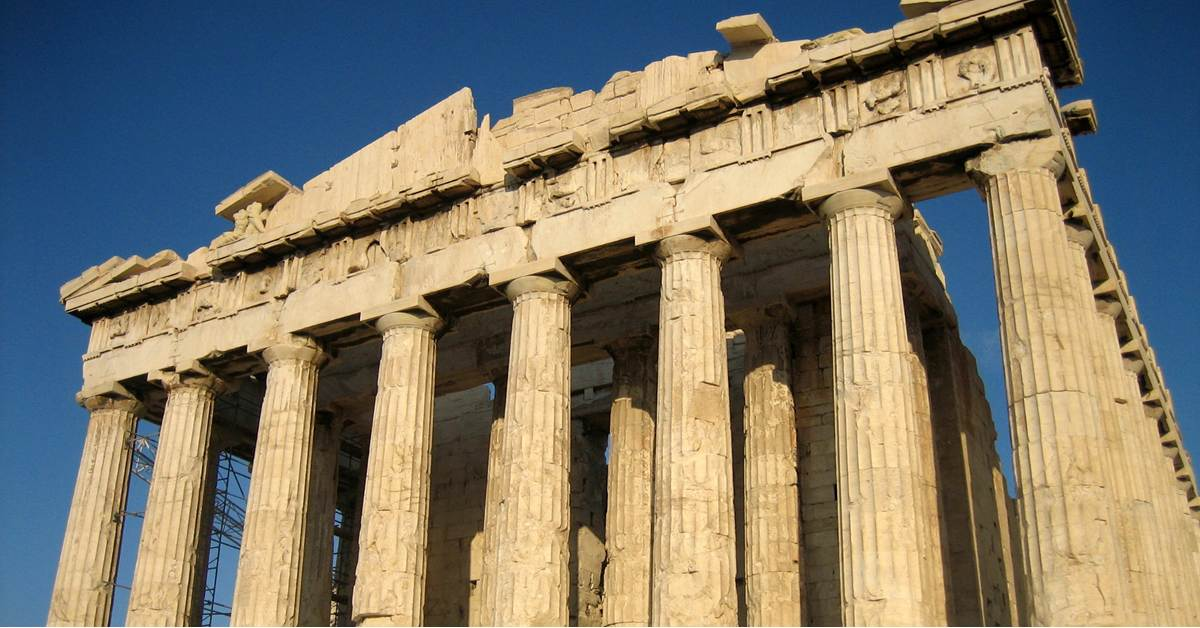The key requirement in successful options trading strategies involves understanding and implementing options pricing models. In this post, we will get a brief understanding about Greeks in options which will help in creating and understanding the pricing models.

Before we start understanding Greeks in options, it is important to get a hang of properties of option contracts. We recommend you read the basic concepts here if you are already not familiar with options. Additionally, there are a few other properties about options which you should know before we delve into the options Greeks.

### Option Pricing is based on two types of values

Intrinsic Value of an option

When the call option stock price is above strike price or when put option stock price is below the strike price, the option is said to be “In-The-Money (ITM)”, i.e. it has an intrinsic value. On the other hand, “Out of the money (OTM)” options have no intrinsic value. For OTM call options, stock price is below strike price and for OTM put options; stock price is above strike price. The price of these options consists entirely of time value.

Time Value of an option

If you subtract the amount of intrinsic value from an option price, you’re left with the time value. It is based on the time to expiration.

### Introduction to Greeks in Options

Greeks are the risk measures associated with various positions in option trading. The common ones are delta, gamma, theta and vega.

With the change in prices or volatility of the underlying stock, you need to know how your option pricing would be affected. Greeks in options help us understand how the various factors such as prices, time to expiry, volatility affect the option pricing.

1. Delta measures the sensitivity of an option’s price to a change in the price of the underlying stock. Simply put, delta is that options greek which tells you how much money a stock option will rise or drop in value with a \$1 rise or drop in the underlying stock which also translates to the amount of profit you will make when the underlying stock rises. Delta is dependent on underlying price, time to expiry and volatility.
2. Gamma measures the exposure of the option delta to the movement of the underlying stock price. Just like delta is the rate of change of option’s price with respect to underlying stock’s price; gamma is the rate of change of delta with respect to underlying stock’s price. Hence, gamma is called the second order derivative.
3. Theta measures the exposure of the option price to the passage of time. It measures the rate at which options price, especially in terms of the time value, changes or decreases as the time to expiry is approached.
4. Vega measures the exposure of the option price to changes in volatility of the underlying. Generally, options are more expensive for higher volatility. So, if the volatility goes up, the price of option might go up to and vice-versa.

### Black-Scholes-Merton Formula for Option Pricing

The formula for the Black-Scholes  option pricing model is given as: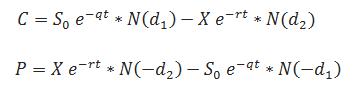Where, C is the price of the call option and P represents the price of a put option.

So is the underlying price, X is the strike price, σ represents volatility, r is the continuously compounded risk-free interest rate, t is the time to expiration, and q is the continuously compounded dividend yield. N(x) is the standard normal cumulative distribution function. The formulas for d1 and d2 are given as: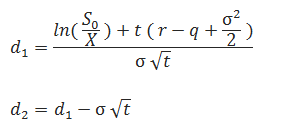To calculate the Greeks in option we use the Black-Scholes option pricing model. Delta and Gamma are calculated as: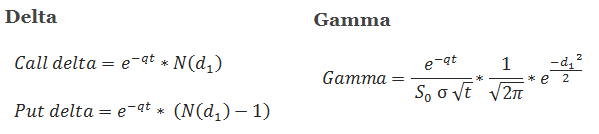Example - In the example below, we have used the determinants of the BS model to compute the Greeks in options.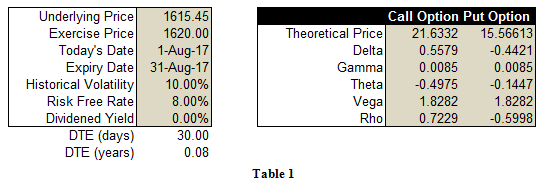At an underlying price of 1615.45 the price of a call option is 21.6332. If we were to increase the price of the underlying by Rs. 1, the change in the price of the call, put and values of the Greeks in the option is as given below.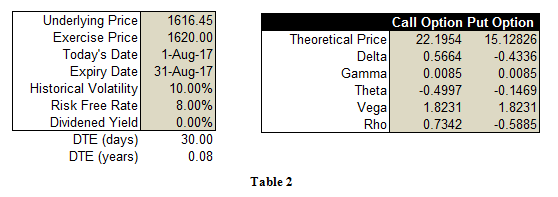As can be observed, the Delta of the call option in the first table was 0.5579. Hence, given the definition of delta, we can expect the price of the call option to increase approximately by this value when the price of the underlying increases by Rs.1. The new price of the call option is 22.1954 which is

21.6332 + 0.5579 ~ 22.1954

22.1911 ~ 22.1954

Let’s move to Gamma, another Greek in option  If you observe the value of Gamma in both the tables, it is the same for both call and put option contracts since it has the same formula for the both option types. If you are long the options, then you would prefer having a higher gamma and if you are short then you would be looking for a low gamma. Thus, if an options trader is having a net-long options position then he will aim to maximize the gamma, whereas in case of a net-short position he will try to minimize the gamma value.

The third Greek, Theta has different formulas for both call and put options. These are given below: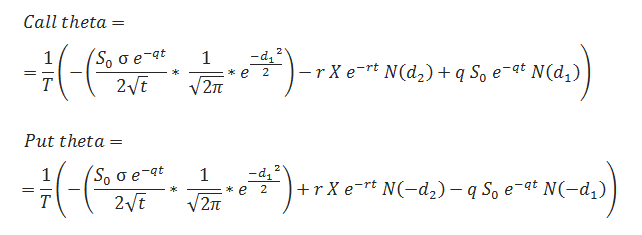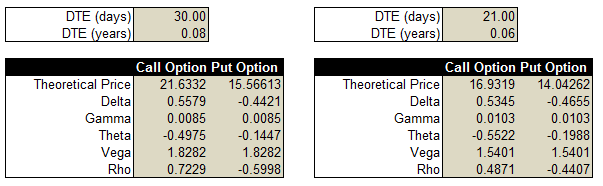In the first table on the LHS, there are 30 days remaining for the option contract to expire. We have a negative theta value of -0.4975 for a long call option position which means that the option trader is running against time. He has to be sure about his analysis in order to profit from trade as time decay will affect this position. This impact of time decay is evident in the table on the RHS where the time left to expiry is now 21 days with other factors remaining the same. As a result, the value of the call option has fallen from 21.6332 to 16.9319. If an options trader wants to profit from the time decay property, he can sell options instead of going long which will result in a positive theta.

We just discussed how some of the individual Greeks in option impact option pricing. However, it is very essential to understand the combined behavior of Greeks on an options position to truly profit from your options position.

Options pricing is a highly mathematical and complex area of study. In the videos below, you can get a glimpse of the discussion held at a seminar at Narsee Monjee Institute of Management Studies between final year students of MBA graduates majoring in Finance and our Options faculty member, Mr. Rajib Ranjan Borah.

#### Highest Gamma for At-the money (ATM) option

Among the three instruments, at-the-money (ATM), out-of-the-money (OTM) and in-the-money (ITM); at the money (ATM) has the highest gamma. Watch the video to understand why! Write in the comments section below if you have any further doubts!